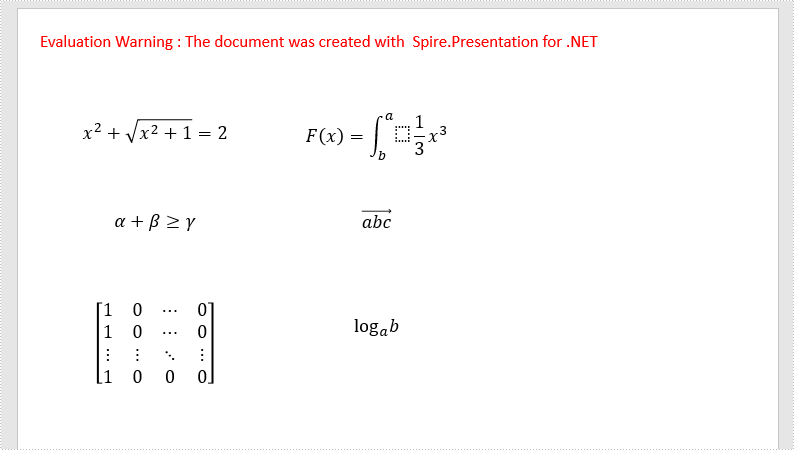## C#/VB.NET: Add Math Equations to PowerPoint using LaTeX Code

Wednesday, 22 September 2021 06:39 Written by  support iceblue
Rate this item

LaTeX is a powerful tool to typeset mathematical equations. It supports plenty of mathematical symbols and notations to create mathematical equations, for instance, fractions, integrals and more.

Spire.Presentation API provides developers with the ability to create and add mathematical equations to PowerPoint shape using LaTeX code. The following steps demonstrate how to achieve this function using Spire.Presentation:

• Create a Presentation instance.
• Get the reference of a slide by using its index.
• Use ShapeList.AppendShape method to add a shape to the first slide.
• Use ParagraphCollection.AddParagraphFromLatexMathCode(string) method to create a mathematical equation from LaTeX code and add it to the shape.
• Save the result document using Presentation.SaveToFile(string, FileFormat) method.

The following code shows how to add mathematical equations to PowerPoint in C#.

using Spire.Presentation;
using System.Drawing;

namespace MathEquations
{
class Program
{
static void Main(string[] args)
{
//The LaTeX codes
string latexCode1 = @"x^{2} + \sqrt{x^{2}+1}=2";
string latexCode2 = @"F(x) &= \int^a_b \frac{1}{3}x^3";
string latexCode3 = @"\alpha + \beta  \geq \gamma";
string latexCode4 = @"\overrightarrow{abc}";
string latexCode5 = @"\begin{bmatrix} 1 & 0 & \cdots & 0\\ 1 & 0 & \cdots & 0\\ \vdots & \vdots & \ddots & \vdots\\ 1 & 0 & 0 & 0 \end{bmatrix}";
string latexCode6 = @"\log_a{b}";

//Create a Presentation instance
Presentation ppt = new Presentation();

//Get the first slide by using its index
ISlide slide = ppt.Slides;

//Add a shape to the slide
IAutoShape shape = slide.Shapes.AppendShape(ShapeType.Rectangle, new RectangleF(30, 100, 200, 30));
shape.TextFrame.Paragraphs.Clear();
//Add a math equation to the shape using the LaTeX code

//Add a shape to the slide
shape = slide.Shapes.AppendShape(ShapeType.Rectangle, new RectangleF(240, 100, 200, 40));
shape.TextFrame.Paragraphs.Clear();
//Add a math equation to the shape using the LaTeX code

//Add a shape to the slide
shape = slide.Shapes.AppendShape(ShapeType.Rectangle, new RectangleF(30, 180, 200, 40));
shape.TextFrame.Paragraphs.Clear();
//Add a math equation to the shape using the LaTeX code

//Add a shape to the slide
shape = slide.Shapes.AppendShape(ShapeType.Rectangle, new RectangleF(240, 180, 200, 40));
shape.TextFrame.Paragraphs.Clear();
//Add a math equation to the shape using the LaTeX code

//Add a shape to the slide
shape = slide.Shapes.AppendShape(ShapeType.Rectangle, new RectangleF(30, 280, 200, 70));
shape.TextFrame.Paragraphs.Clear();
//Add a math equation to the shape using the LaTeX code

//Add a shape to the slide
shape = slide.Shapes.AppendShape(ShapeType.Rectangle, new RectangleF(240, 280, 200, 40));
shape.TextFrame.Paragraphs.Clear();
//Add a math equation to the shape using the LaTeX code

for (int i = 0; i < slide.Shapes.Count; i++)
{
slide.Shapes[i].Fill.FillType = Spire.Presentation.Drawing.FillFormatType.None;
slide.Shapes[i].Line.FillType = Spire.Presentation.Drawing.FillFormatType.None;
}

//Save the result document
ppt.SaveToFile("MathEquations.pptx", FileFormat.Pptx2013);
}
}
}


The following code shows how to add mathematical equations to PowerPoint in VB.NET.

Imports Spire.Presentation
Imports System.Drawing

Namespace MathEquations
Friend Class Program
Private Shared Sub Main(ByVal args As String())
'The LaTeX codes
Dim latexCode1 As String = "x^{2} + \sqrt{x^{2}+1}=2"
Dim latexCode2 As String = "F(x) &= \int^a_b \frac{1}{3}x^3"
Dim latexCode3 As String = "\alpha + \beta  \geq \gamma"
Dim latexCode4 As String = "\overrightarrow{abc}"
Dim latexCode5 As String = "\begin{bmatrix} 1 & 0 & \cdots & 0\\ 1 & 0 & \cdots & 0\\ \vdots & \vdots & \ddots & \vdots\\ 1 & 0 & 0 & 0 \end{bmatrix}"
Dim latexCode6 As String = "\log_a{b}"

'Create a Presentation instance
Dim ppt As Presentation = New Presentation()

'Get the first slide by using its index
Dim slide As ISlide = ppt.Slides(0)

'Add a shape to the slide
Dim shape As IAutoShape = slide.Shapes.AppendShape(ShapeType.Rectangle, New RectangleF(30, 100, 200, 30))
shape.TextFrame.Paragraphs.Clear()
'Add a math equation to the shape using the LaTeX code

'Add a shape to the slide
shape = slide.Shapes.AppendShape(ShapeType.Rectangle, New RectangleF(240, 100, 200, 40))
shape.TextFrame.Paragraphs.Clear()
'Add a math equation to the shape using the LaTeX code

'Add a shape to the slide
shape = slide.Shapes.AppendShape(ShapeType.Rectangle, New RectangleF(30, 180, 200, 40))
shape.TextFrame.Paragraphs.Clear()
'Add a math equation to the shape using the LaTeX code

'Add a shape to the slide
shape = slide.Shapes.AppendShape(ShapeType.Rectangle, New RectangleF(240, 180, 200, 40))
shape.TextFrame.Paragraphs.Clear()
'Add a math equation to the shape using the LaTeX code

'Add a shape to the slide
shape = slide.Shapes.AppendShape(ShapeType.Rectangle, New RectangleF(30, 280, 200, 70))
shape.TextFrame.Paragraphs.Clear()
'Add a math equation to the shape using the LaTeX code

'Add a shape to the slide
shape = slide.Shapes.AppendShape(ShapeType.Rectangle, New RectangleF(240, 280, 200, 40))
shape.TextFrame.Paragraphs.Clear()
'Add a math equation to the shape using the LaTeX code

For i As Integer = 0 To slide.Shapes.Count - 1
slide.Shapes(i).Fill.FillType = Spire.Presentation.Drawing.FillFormatType.None
slide.Shapes(i).Line.FillType = Spire.Presentation.Drawing.FillFormatType.None
Next

'Save the result document
ppt.SaveToFile("MathEquations.pptx", FileFormat.Pptx2013)
End Sub
End Class
End Namespace


The following is the output document after adding mathematical equations:### Apply for a Temporary License

If you'd like to remove the evaluation message from the generated documents, or to get rid of the function limitations, please request a 30-day trial license for yourself.